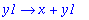Function: vnapply - Make a function from an expression and arguments (replacement for unapply)

Calling Sequence:

vnapply(expr, vars);

Parameters:

expr - any expression

vars - one or more variables (may be any non-constant expressions)

Description:

The result of vnapply(expr,x) is a function which, when applied to x , returns the original expression expr .

The "variables" vars may be arbitrary non-constant expressions, but these will only be recognized in expr if they occur there as operands. This is the same behaviour as occurs in subs . Thus vnapply(sin(x+y),x+y) will work, but vnapply(sin(x+y+z),x+y) will not.

This is a replacement for unapply , correcting several problems with that procedure:

- Sometimes unapply returns a result of the form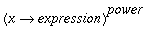or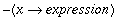, vnapply does not. In these cases, the result of unapply will not work under evalhf , while the result of vnapply will.

- vnapply works with expressions that are arrays (including vectors and matrices) or tables, which unapply does not..

- vnapply works with expressions containing derivatives (converting them to D notation), while in many cases unapply does not.

This function is part of the Maple Advisor Database library, and must be loaded before use by the command readlib(vnapply); .

Examples:

> f1 := unapply(x^2,x); f2 := vnapply(x^2,x);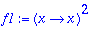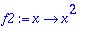> f1 := unapply(-cos(x+1),x); f2 := vnapply(-cos(x+1),x);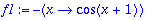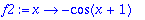> f1:= unapply(diff(g(x,y),x,y),x,y);
f2:= vnapply(diff(g(x,y),x,y),x,y);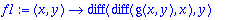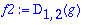> f1(3,4); f2(3,4);

Error, (in f1) wrong number (or type) of parameters in function diff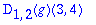> V:= vector([x,x^2]): f1:= unapply(V,x);
f2:= vnapply(V,x);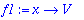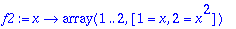> f1(u),f2(u);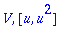> unapply(x+g(z),g(z));

Error, (in unapply) variables must be unique and of type name

> vnapply(x+g(z),g(z));